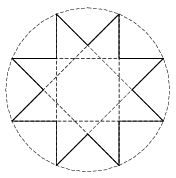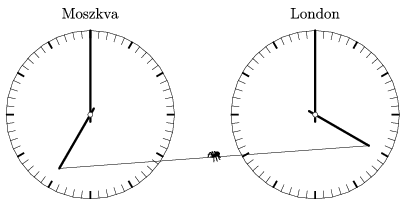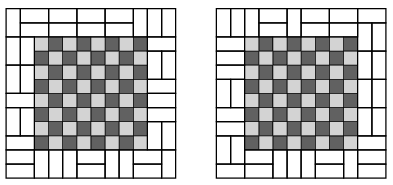Mathematical and Physical Journal
for High Schools
Issued by the MATFUND Foundation
 Already signed up? New to KöMaL?

# KöMaL Problems in Mathematics, December 2017

Show/hide problems of signs:## Problems with sign 'K'

Deadline expired on January 10, 2018.

K. 565. Arthur Dumpling the fat bird (a popular Hungarian cartoon character) plans to make a new year's resolution on 31 December 2017. Starting with 1 January 2018, he would go on a special slimming diet. On each day, he needs to start by calculating how many bars of chocolate he is allowed to eat that day. He considers the number of the current day in the year 2018. If the day number is even then he may eat as many bars of chocolate as on the day with half the current day number. (For example, on the 26th day of the year he is allowed to eat as many bars as on day 13.) If the day number is odd and greater than 1, then he may eat one fewer as he will be allowed to eat on the following day. The slimming diet ends on 30 December. Given that Arthur will eat 3 bars of chocolate on 9 January, how many bars will he eat on 24 December 2018?

(6 pont)

solution (in Hungarian), statistics

K. 566. The lengths of the sides of a quadrilateral $\displaystyle ABCD$ are $\displaystyle AB = 8$ cm, $\displaystyle BC= 7$ cm, $\displaystyle CD = 6$ cm and $\displaystyle DA = 5$ cm. The inscribed circles of triangles $\displaystyle BCD$ and $\displaystyle ABD$ touch diagonal $\displaystyle BD$ at points $\displaystyle E$ and $\displaystyle F$, respectively. What is the length of the line segment $\displaystyle EF$?

(6 pont)

solution (in Hungarian), statistics

K. 567. Find all positive integers $\displaystyle n$ less than 1000 whose square ends in $\displaystyle n$.

(6 pont)

solution (in Hungarian), statistics

K. 568. $\displaystyle a)$ Find a set of four different prime numbers less than 50 such that the sum of any three of them is also a prime.

$\displaystyle b)$ Are there five different positive primes such that the sum of any three of them is also a prime?

(6 pont)

solution (in Hungarian), statistics

K. 569. Determine the four-digit positive integer $\displaystyle \overline{abcd}$ for which $\displaystyle \overline{abcd} =a^{a}+b^{b}+c^{c}+d^{d}$.

(6 pont)

solution (in Hungarian), statistics

K. 570. Five bystanders are trying predict the outcome of a football game. They make the following statements about the two teams, Team Fox and Team Wolf.

Aron: Team Fox will score more than Team Wolf. Team Wolf will score at least 2 goals.

Bob: The game will not be a draw. Team Fox will score 1 goal.

Cecil: The winning team will win by 2 goals. At half-time, Team Wolf will have scored more.

Dan: Team Wolf will not score any goal. Team Fox will win the game.

Eve: In the second half of the game, Team Fox will score twice as many goals as Team Wolf. The game will be a draw.

We know that finally there was no own goal during the game, and everyone had one true and one false statement in the above list. What is the final result of the game?

(6 pont)

solution (in Hungarian), statistics## Problems with sign 'C'

Deadline expired on January 10, 2018.

C. 1448. Solve the following equation on the set of positive integers:

$\displaystyle \left[\frac{2017}{x}\right]+\left[\frac{2018}{x+1}\right]=230,$

where $\displaystyle [a]$ denotes the greatest integer that is not greater than $\displaystyle a$.

Proposed by Zs. M. Tatár, Felsőgöd

(5 pont)

solution (in Hungarian), statistics

C. 1449. A regular eight-pointed star is inscribed in a unit circle as shown in the figure. How long is the perimeter of the star?(5 pont)

solution (in Hungarian), statistics

C. 1450. In base $\displaystyle n$ notation, where $\displaystyle n>3$, a number is divisible by 3 if and only if the sum of its digits is divisible by 3. Determine all such $\displaystyle n$.

(5 pont)

solution (in Hungarian), statistics

C. 1451. Where does the curve of equation $\displaystyle y=x|x|-2x+3$ intersect the $\displaystyle x$ axis? Find the location and type of local extrema of this curve, and determine the corresponding $\displaystyle y$ coordinates.

(5 pont)

solution (in Hungarian), statistics

C. 1452. A trapezium is inscribed in a circle of radius 13 cm. The distance of the diagonals of the trapezium from the centre of the circle is 5 cm. What is the maximum possible area of the trapezium?

(5 pont)

solution (in Hungarian), statistics

C. 1453. A $\displaystyle 3\times3$ square lattice contains twelve grid line segments of unit length in its interior. Four out of the twelve line segments are selected at random. What is the probability that the selected line segments divide the square into at least two parts?

(5 pont)

solution (in Hungarian), statistics

C. 1454. There are two identical clocks at the reception of a hotel. One clock shows the time in London, and the other shows the time in Moscow. A spider has made a web between the hour hands of the two clocks. While the thread is stretching elastically, the spider always stays at the midpoint. What will be the path described by the spider during the course of 24 hours? (The time lag between London and Moscow is 3 hours.)(5 pont)

solution (in Hungarian), statistics## Problems with sign 'B'

Deadline expired on January 10, 2018.

B. 4912. Prove that the equation $\displaystyle 5x^2-4y^2=2017$ has no integer solution.

(3 pont)

solution (in Hungarian), statistics

B. 4913. The diagonal $\displaystyle AC$ bisects the angle lying at vertex $\displaystyle A$ of a cyclic quadrilateral $\displaystyle ABCD$. Let $\displaystyle E$ denote a point on the extension of side $\displaystyle AD$ beyond $\displaystyle D$. Show that $\displaystyle CE=CA$ holds if and only if $\displaystyle DE=AB$.

Bulgarian problem

(3 pont)

solution (in Hungarian), statistics

B. 4914. Let $\displaystyle p(x)$ be a polynomial of integer coefficients such that there exist $\displaystyle 4$ distinct integers $\displaystyle a$, $\displaystyle b$, $\displaystyle c$, $\displaystyle d$ with $\displaystyle p(a)=p(b)=p(c)=p(d)=2000$.

$\displaystyle a)$ Prove that there is no integer $\displaystyle x_0$ for which $\displaystyle p(x_0)=2017$.

$\displaystyle b)$ Find a polynomial $\displaystyle p(x)$ that meets the above requirement, and find an integer $\displaystyle x_1$ for which $\displaystyle p(x_1)=2018$.

(4 pont)

solution (in Hungarian), statistics

B. 4915. Given any points $\displaystyle A_1$, $\displaystyle A_2$, $\displaystyle A_3$, $\displaystyle A_4$, $\displaystyle A_5$ and $\displaystyle P$ in the plane, let $\displaystyle k_i$ denote the number of ways it is possible to select $\displaystyle i$ points out of $\displaystyle A_1$, $\displaystyle A_2$, $\displaystyle A_3$, $\displaystyle A_4$, $\displaystyle A_5$ such that the convex hull of the selected points should contain $\displaystyle P$. Show that $\displaystyle k_3=k_4$.

(5 pont)

solution (in Hungarian), statistics

B. 4916. Let $\displaystyle P(a,b,c)$ be a fixed point in the 3D Cartesian coordinate system. Let $\displaystyle a,b,c>0$ and let $\displaystyle O$ denote the origin. Let $\displaystyle S$ be a plane passing through $\displaystyle P$ that intersects the positive coordinate axes at the points $\displaystyle X$, $\displaystyle Y$ and $\displaystyle Z$. Show that the volume of tetrahedron $\displaystyle OXYZ$ is a minimum if and only if $\displaystyle P$ is the centroid of triangle $\displaystyle XYZ\triangle$.

(4 pont)

solution (in Hungarian), statistics

B. 4917. Determine all functions $\displaystyle f \colon \big(\mathbb{R} \setminus \{0,1\}\big)\to \mathbb{R}$ for which

$\displaystyle f\left(\frac{x-1}{x}\right)+f\left(\frac{1}{1-x}\right)=4-\frac2x.$

Proposed by B. Kovács, Szatmárnémeti

(5 pont)

solution (in Hungarian), statistics

B. 4918. Given $\displaystyle M$ unit vectors in the space ($\displaystyle M\ge 2$), prove that it is possible to select $\displaystyle M-1$ unit vectors such that the length of their sum is at least unity.

(5 pont)

solution (in Hungarian), statistics

B. 4919. Let $\displaystyle I$ be the centre of the inscribed circle of triangle $\displaystyle ABC$. The inscribed circle touches the sides $\displaystyle BC$, $\displaystyle CA$ and $\displaystyle AB$ at the points $\displaystyle A_1$, $\displaystyle B_1$ and $\displaystyle C_1$, respectively. The lines $\displaystyle AB$ and $\displaystyle A_1B_1$ intersect at point $\displaystyle K$. Show that the circle centred at $\displaystyle K$ and passing through $\displaystyle C_1$, and the inscribed circles of the kites $\displaystyle AC_1IB_1$ and $\displaystyle BA_1IC_1$ have a tangent in common, and that tangent passes through the point $\displaystyle C$.

(6 pont)

solution (in Hungarian), statistics

B. 4920. A frame of width 2 units is drawn around an $\displaystyle 8\times8$ chessboard. In how many different ways is it possible to cover that frame without gaps or overlaps with $\displaystyle 1\times2$ dominoes? (The two arrangements shown in the figure are considered different.)Proposed by S. Róka, Nyíregyháza

(6 pont)

solution (in Hungarian), statistics## Problems with sign 'A'

Deadline expired on January 10, 2018.

A. 710. For which $\displaystyle n$ can we partition a regular $\displaystyle n$-gon into finitely many triangles such that no two triangles share a side?

(Based on a problem of the 2017 Miklós Schweitzer competition)

(5 pont)

solution (in Hungarian), statistics

A. 711. For which pairs $\displaystyle (m,n)$ does there exist an injective function $\displaystyle f\colon \mathbb{R}^2\to\mathbb{R}^2$ under which the image of every regular $\displaystyle m$-gon is a regular $\displaystyle n$-gon. (Note that $\displaystyle m,n\ge 3$, and that by a regular $\displaystyle N$-gon we mean the union of the boundary segments, not the closed polygonal region.)

Proposed by: Sutanay Bhattacharya, Bishnupur, India

(5 pont)

statistics

A. 712. We say that a strictly increasing positive real sequence $\displaystyle a_1,a_2,\dots$ is an elf sequence if for any $\displaystyle c>0$ we can find an $\displaystyle N$ such that $\displaystyle a_n<cn$ for $\displaystyle n=N,N+1,\dots\,.$ Furthermore, we say that $\displaystyle a_n$ is a hat if $\displaystyle a_{n-i}+a_{n+i}<2a_n$ for $\displaystyle 1\le i\le n-1$. Is it true that every elf sequence has infinitely many hats?

(5 pont)

solution, statistics